# NCERT Exemplar Class 11 Chemistry Solutions for Chapter 3 - Classification of Elements and Periodicity in Properties

## NCERT Exemplar Solutions Class 11 Chemistry Chapter 3 – Free PDF Download

The NCERT Exemplar Chemistry Class 11 Chapter 3 Classification of Elements and Periodicity in Properties provides you with questions which are important for the final exam. It also helps you to understand concepts involved in the periodicity of the element. Students are advised to study these exemplars to get a good score in Class 11 and entrance examinations. The NCERT Exemplar Class 11 Chemistry Chapter 3 has questions from the NCERT exemplar book, along with important questions from previous years’ question papers and sample papers. Moreover, the solution is prepared by experts at BYJU’S. This will be helpful in grasping Chemistry Chapter 3 Class 11 notes and solving entrance exam questions.

In this chapter, students will study the historical development of the periodic table and its current status. Further, they will learn about the modern periodic law and how periodic classification takes place. Students will also examine some of the periodic trends in the physical and chemical properties of elements. To help students be thorough with all chapter topics, like elements, Bohr’s model, atomic model, a quantum mechanical model, free NCERT Exemplar for Class 11 Chemistry Chapter 3 – classification of elements and periodicity in properties are available here. The NCERT Exemplar contains detailed answers to all chapter questions, which students can go through and understand what the right solution for a particular question is. This will help them study smartly and be ready to face the annual exam with confidence.

### Download the PDF of NCERT Exemplar Solutions for Class 11 Chemistry Chapter 3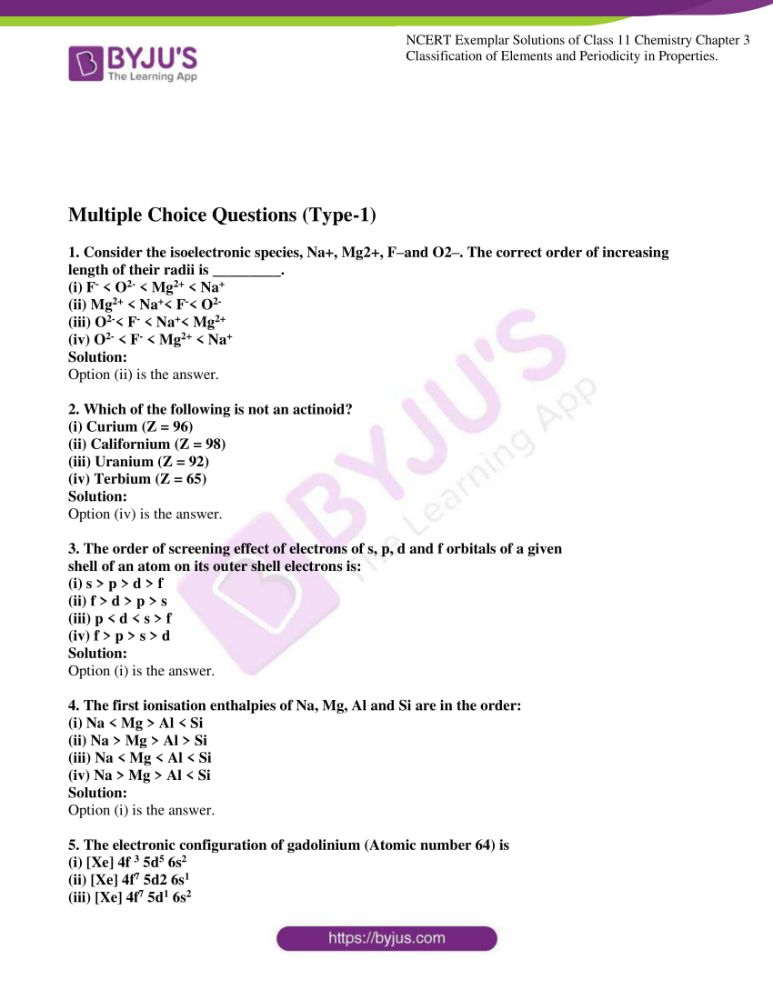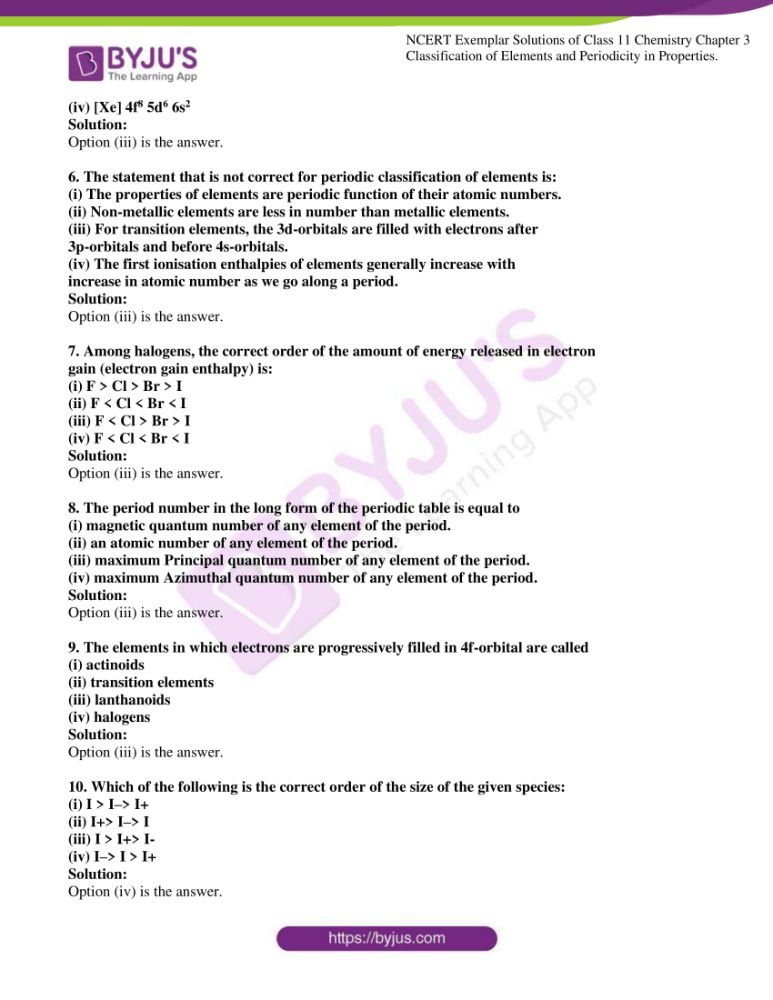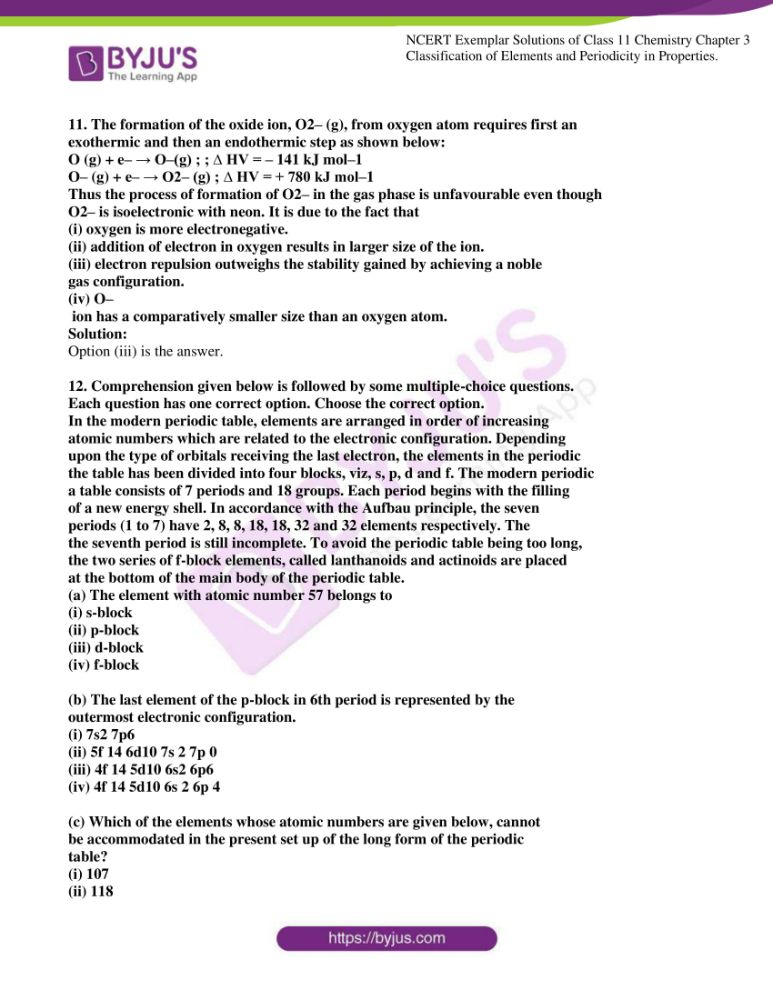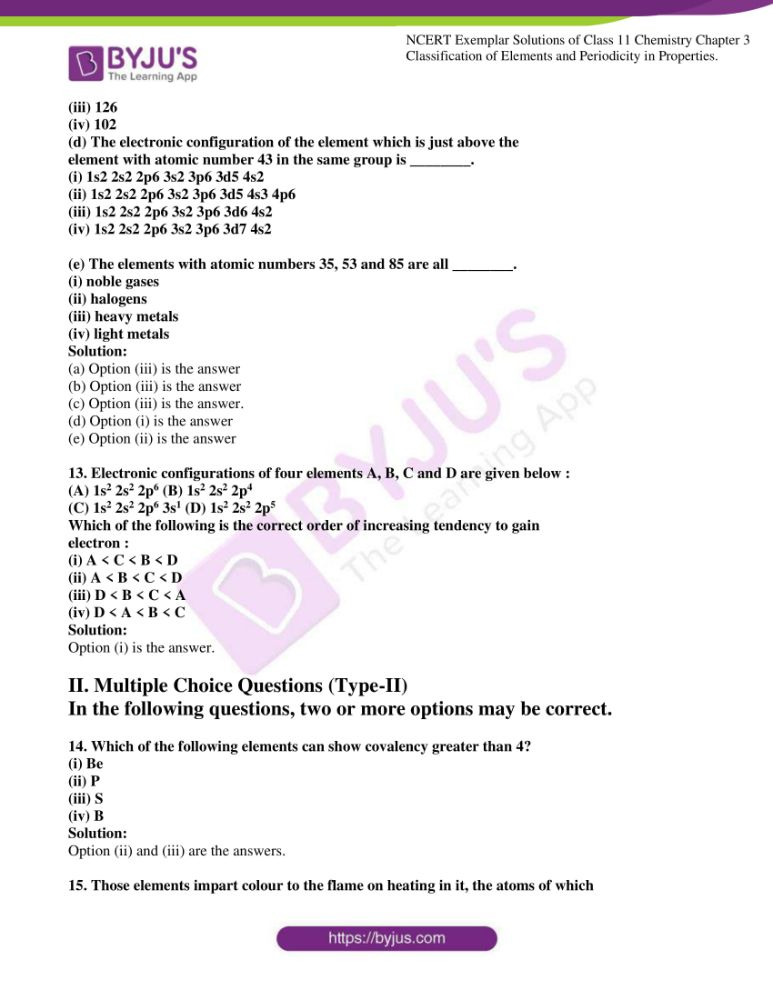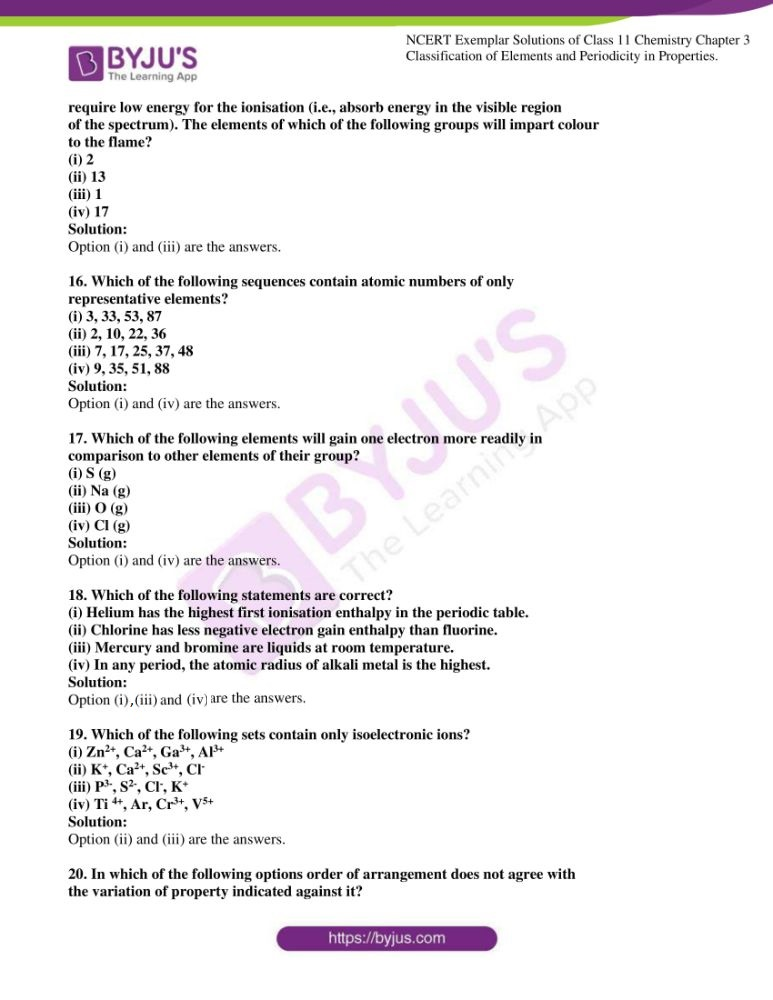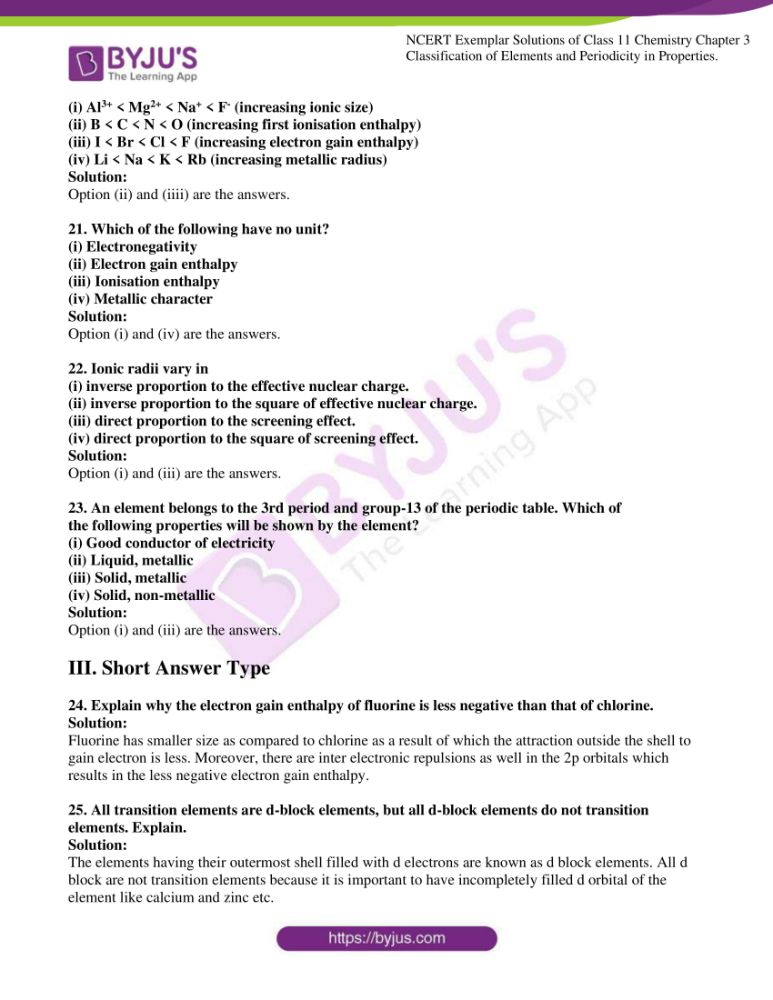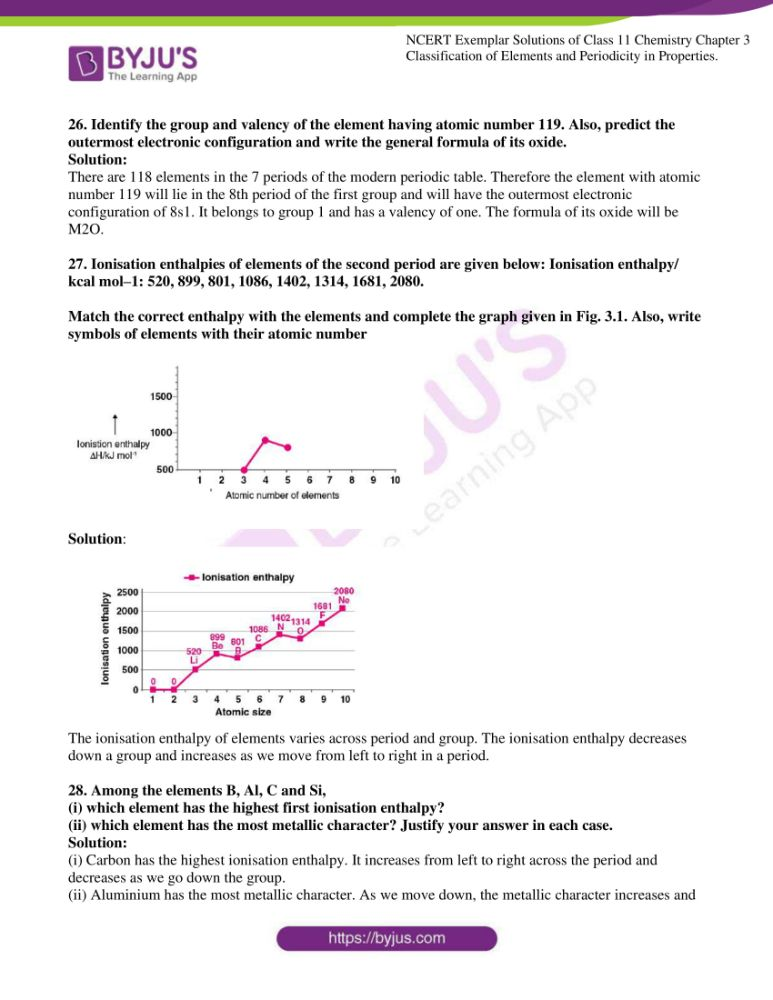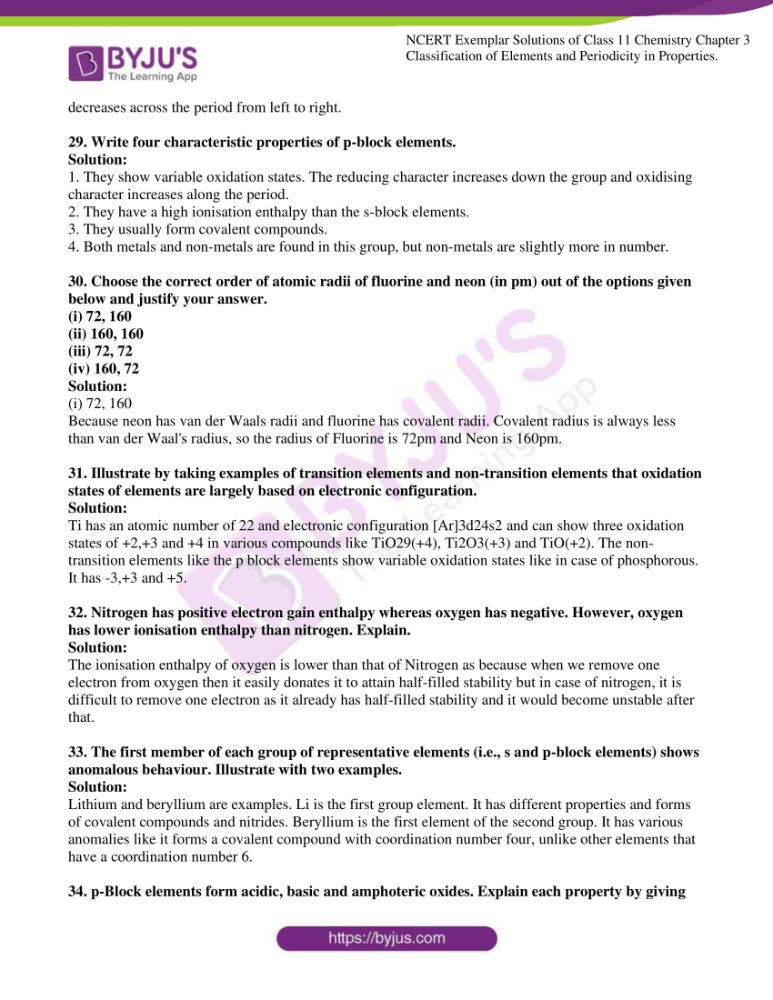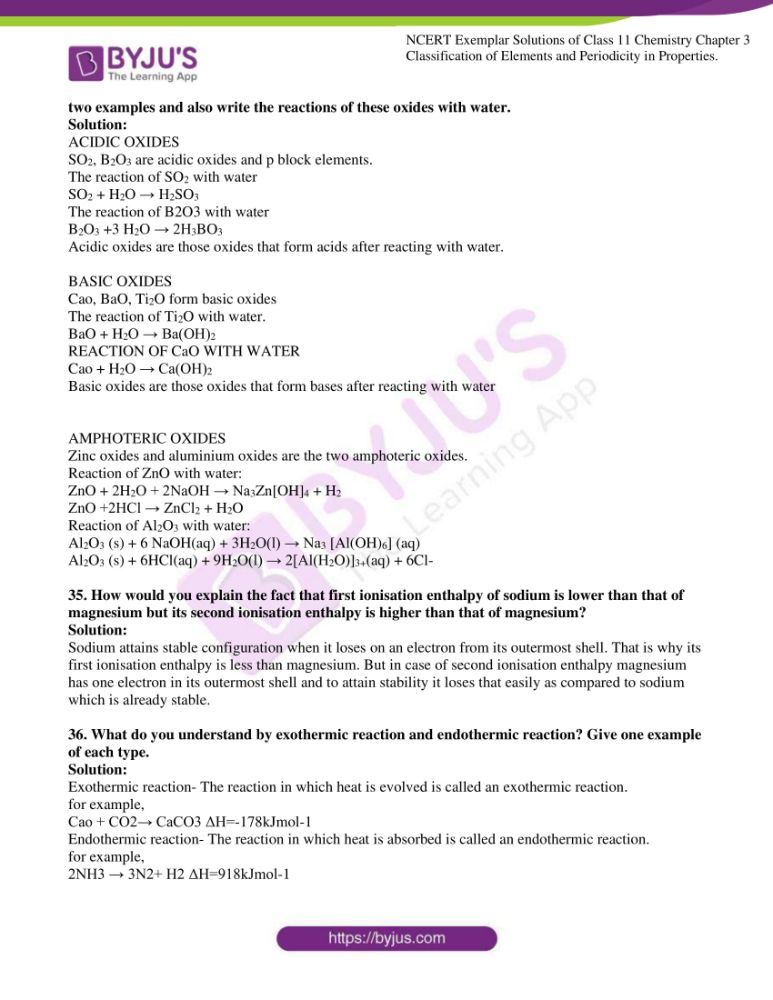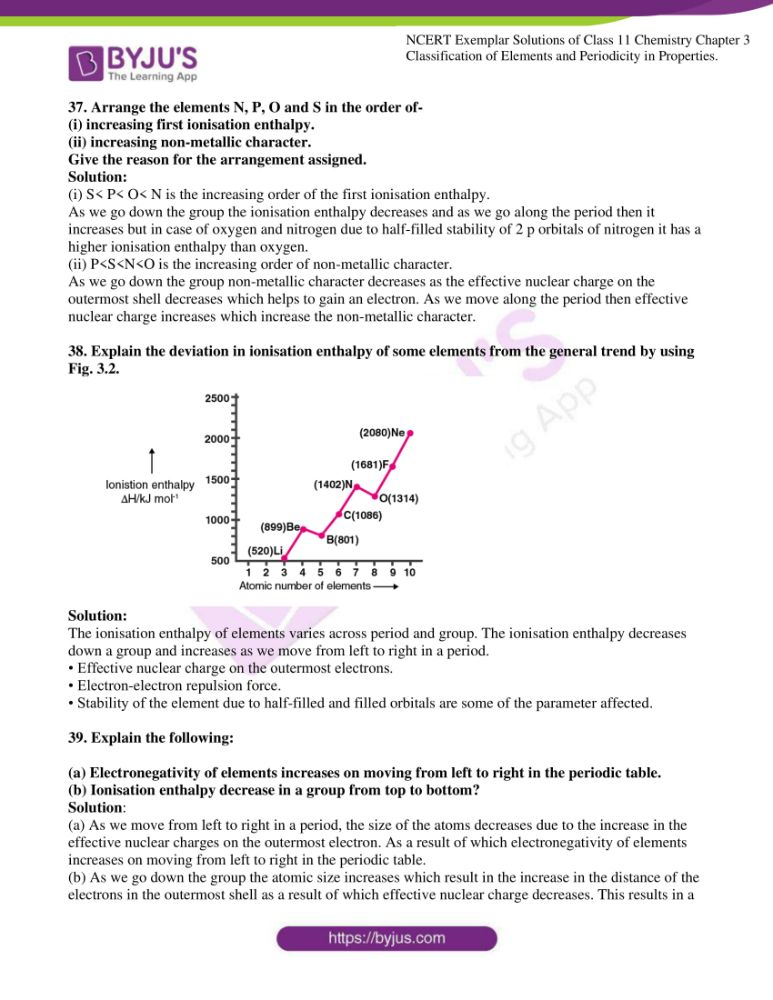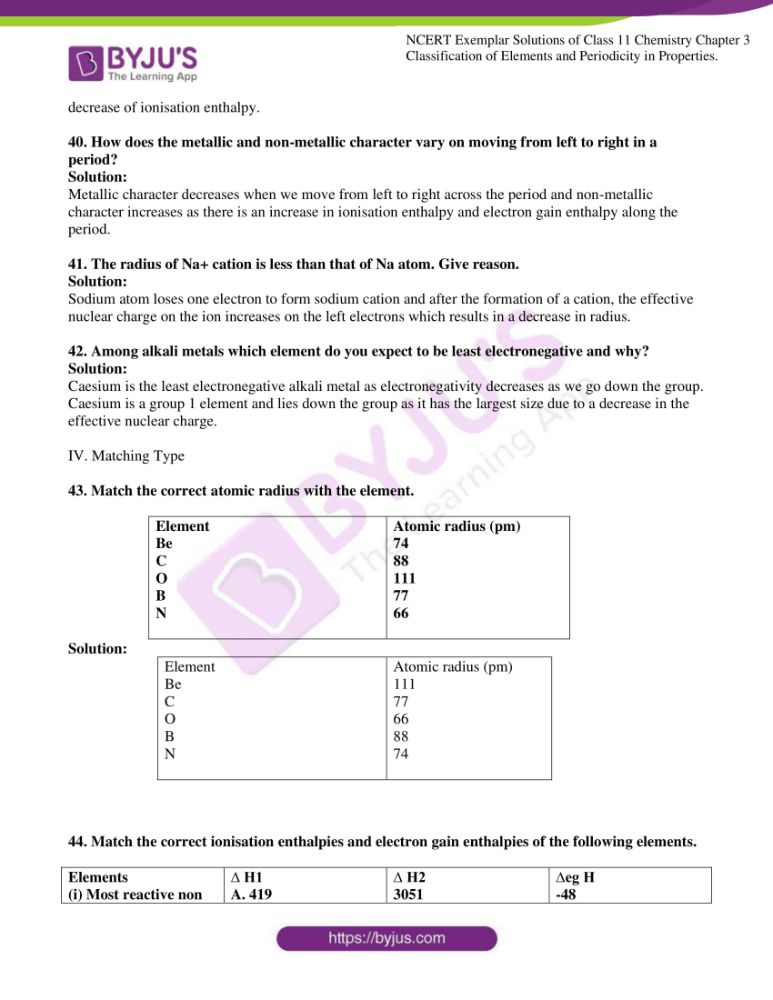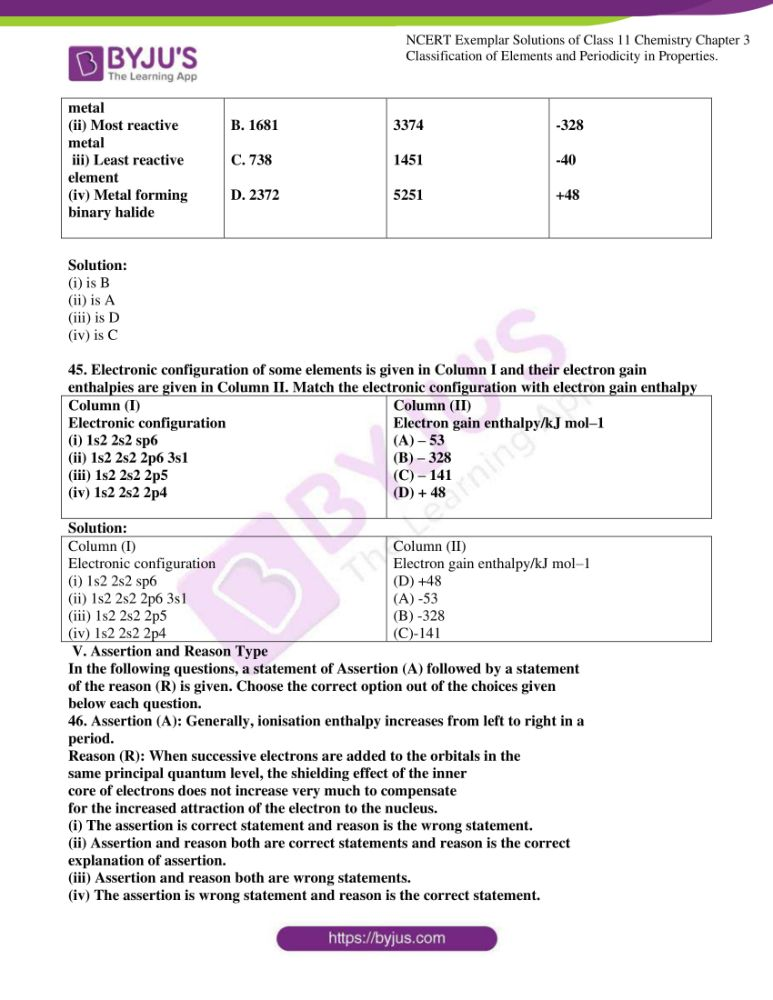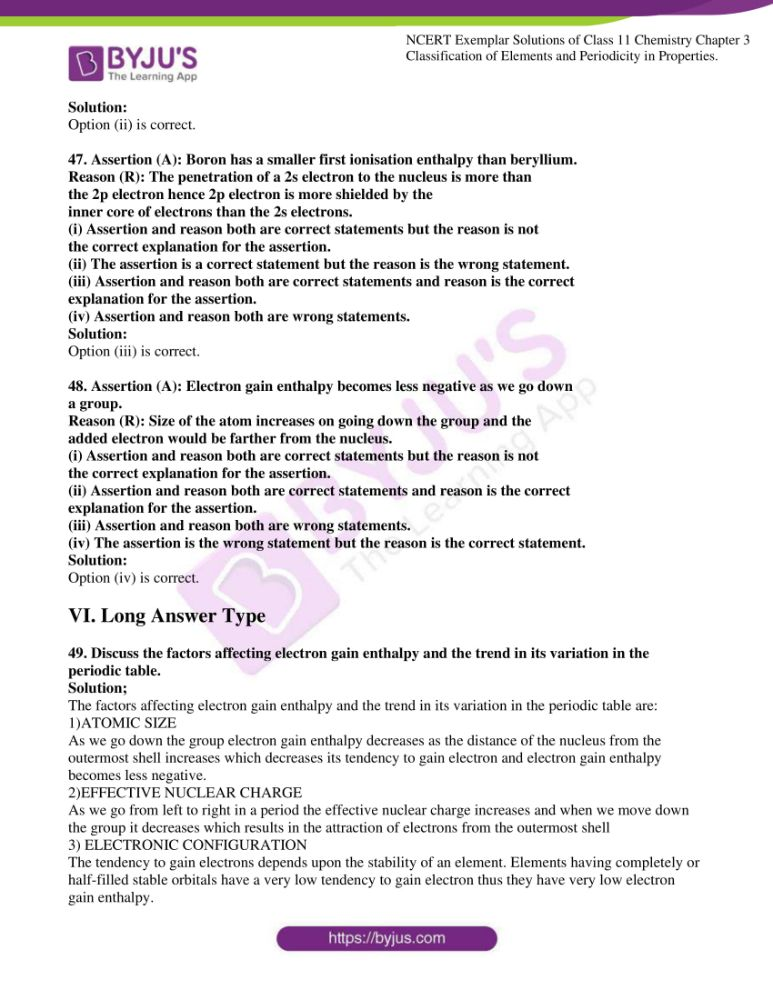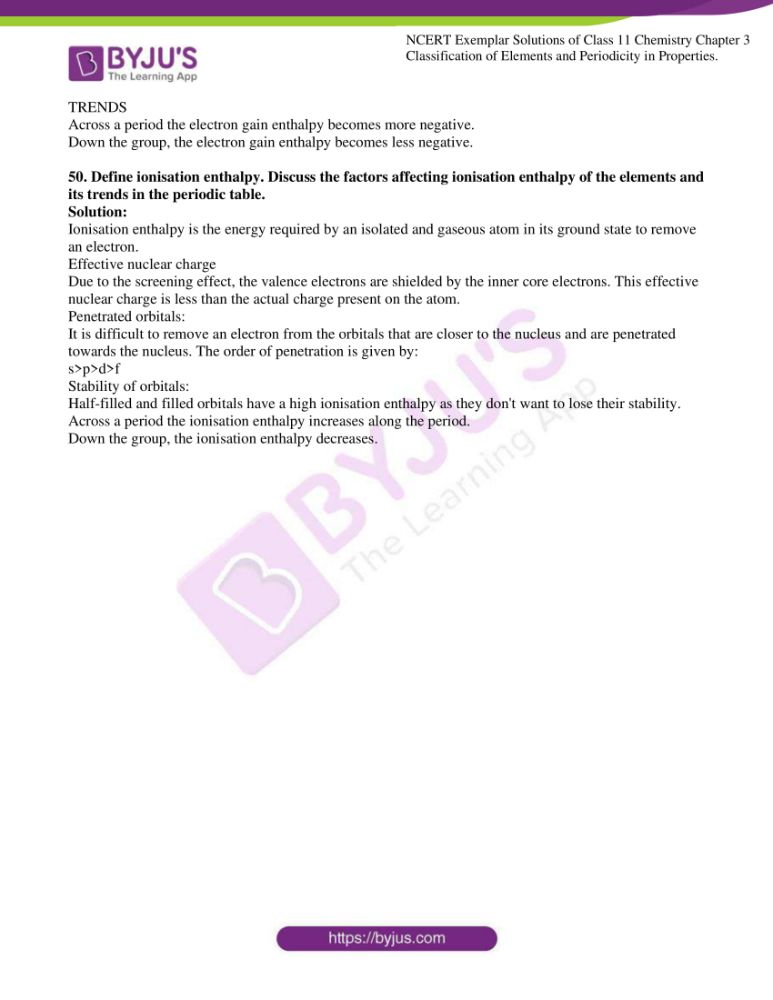### Access Answers to the NCERT Exemplar Class 11 Chemistry Chapter 3

Multiple Choice Questions (Type-1)

1. Consider the isoelectronic species, Na+, Mg2+, F–and O2–. The correct order of increasing length of their radii is _________.

(i) F < O2- < Mg2+ < Na+

(ii) Mg2+ < Na+< F< O2-

(iii) O2-< F < Na+< Mg2+

(iv) O2- < F < Mg2+ < Na+

Solution:

2. Which of the following is not an actinoid?

(i) Curium (Z = 96)

(ii) Californium (Z = 98)

(iii) Uranium (Z = 92)

(iv) Terbium (Z = 65)

Solution:

3. The order of screening effect of electrons of s, p, d and f orbitals of a given

shell of an atom on its outer shell electrons is:

(i) s > p > d > f

(ii) f > d > p > s

(iii) p < d < s > f

(iv) f > p > s > d

Solution:

4. The first ionisation enthalpies of Na, Mg, Al and Si are in the order:

(i) Na < Mg > Al < Si

(ii) Na > Mg > Al > Si

(iii) Na < Mg < Al < Si

(iv) Na > Mg > Al < Si

Solution:

5. The electronic configuration of gadolinium (Atomic number 64) is

(i) [Xe] 4f 3 5d5 6s2

(ii) [Xe] 4f7 5d2 6s1

(iii) [Xe] 4f7 5d1 6s2

(iv) [Xe] 4f8 5d6 6s2

Solution:

6. The statement that is not correct for periodic classification of elements is:

(i) The properties of elements are periodic function of their atomic numbers.

(ii) Non-metallic elements are less in number than metallic elements.

(iii) For transition elements, the 3d-orbitals are filled with electrons after

3p-orbitals and before 4s-orbitals.

(iv) The first ionisation enthalpies of elements generally increase with

increase in atomic number as we go along a period.

Solution:

7. Among halogens, the correct order of the amount of energy released in electron

gain (electron gain enthalpy) is:

(i) F > Cl > Br > I

(ii) F < Cl < Br < I

(iii) F < Cl > Br > I

(iv) F < Cl < Br < I

Solution:

8. The period number in the long form of the periodic table is equal to

(i) magnetic quantum number of any element of the period.

(ii) an atomic number of any element of the period.

(iii) maximum Principal quantum number of any element of the period.

(iv) maximum Azimuthal quantum number of any element of the period.

Solution:

9. The elements in which electrons are progressively filled in 4f-orbital are called

(i) actinoids

(ii) transition elements

(iii) lanthanoids

(iv) halogens

Solution:

10. Which of the following is the correct order of the size of the given species:

(i) I > I–> I+

(ii) I+> I–> I

(iii) I > I+> I-

(iv) I–> I > I+

Solution:

11. The formation of the oxide ion, O2– (g), from oxygen atom requires first an

exothermic and then an endothermic step as shown below:

O (g) + e– → O–(g) ; ; ∆ HV = – 141 kJ mol–1

O– (g) + e– → O2– (g) ; ∆ HV = + 780 kJ mol–1

Thus the process of formation of O2– in the gas phase is unfavourable even though

O2– is isoelectronic with neon. It is due to the fact that

(i) oxygen is more electronegative.

(ii) addition of electron in oxygen results in larger size of the ion.

(iii) electron repulsion outweighs the stability gained by achieving a noble

gas configuration.

(iv) O–

ion has a comparatively smaller size than an oxygen atom.

Solution:

12. Comprehension given below is followed by some multiple-choice questions.

Each question has one correct option. Choose the correct option.

In the modern periodic table, elements are arranged in order of increasing

atomic numbers which are related to the electronic configuration. Depending

upon the type of orbitals receiving the last electron, the elements in the periodic

the table has been divided into four blocks, viz, s, p, d and f. The modern periodic

table consists of 7 periods and 18 groups. Each period begins with the filling

of a new energy shell. In accordance with the Aufbau principle, the seven

periods (1 to 7) have 2, 8, 8, 18, 18, 32 and 32 elements respectively. The

seventh period is still incomplete. To avoid the periodic table being too long,

the two series of f-block elements, called lanthanoids and actinoids are placed

at the bottom of the main body of the periodic table.

(a) The element with atomic number 57 belongs to

(i) s-block

(ii) p-block

(iii) d-block

(iv) f-block

(b) The last element of the p-block in 6th period is represented by the

outermost electronic configuration.

(i) 7s2 7p6

(ii) 5f 14 6d10 7s 2 7p 0

(iii) 4f 14 5d10 6s2 6p6

(iv) 4f 14 5d10 6s 2 6p 4

(c) Which of the elements whose atomic numbers are given below, cannot

be accommodated in the present set up of the long form of the periodic

table?

(i) 107

(ii) 118

(iii) 126

(iv) 102

(d) The electronic configuration of the element which is just above the

element with atomic number 43 in the same group is ________.

(i) 1s2 2s2 2p6 3s2 3p6 3d5 4s2

(ii) 1s2 2s2 2p6 3s2 3p6 3d5 4s3 4p6

(iii) 1s2 2s2 2p6 3s2 3p6 3d6 4s2

(iv) 1s2 2s2 2p6 3s2 3p6 3d7 4s2

(e) The elements with atomic numbers 35, 53 and 85 are all ________.

(i) noble gases

(ii) halogens

(iii) heavy metals

(iv) light metals

Solution:

(a) Option (iii) is the answer

(b) Option (iii) is the answer

(c) Option (iii) is the answer.

(d) Option (i) is the answer

(e) Option (ii) is the answer

13. Electronic configurations of four elements A, B, C and D are given below :

(A) 1s2 2s2 2p6 (B) 1s2 2s2 2p4

(C) 1s2 2s2 2p6 3s1 (D) 1s2 2s2 2p5

Which of the following is the correct order of increasing tendency to gain

electron :

(i) A < C < B < D

(ii) A < B < C < D

(iii) D < B < C < A

(iv) D < A < B < C

Solution:

II. Multiple Choice Questions (Type-II)

In the following questions, two or more options may be correct.

14. Which of the following elements can show covalency greater than 4?

(i) Be

(ii) P

(iii) S

(iv) B

Solution:

Option (ii) and (iii) are the answers.

15. Those elements impart colour to the flame on heating in it, the atoms of which

require low energy for the ionisation (i.e., absorb energy in the visible region

of the spectrum). The elements of which of the following groups will impart colour

to the flame?

(i) 2

(ii) 13

(iii) 1

(iv) 17

Solution:

Option (i) and (iii) are the answers.

16. Which of the following sequences contain atomic numbers of only

representative elements?

(i) 3, 33, 53, 87

(ii) 2, 10, 22, 36

(iii) 7, 17, 25, 37, 48

(iv) 9, 35, 51, 88

Solution:

Option (i) and (iv) are the answers.

17. Which of the following elements will gain one electron more readily in

comparison to other elements of their group?

(i) S (g)

(ii) Na (g)

(iii) O (g)

(iv) Cl (g)

Solution:

Option (i) and (iv) are the answers.

18. Which of the following statements are correct?

(i) Helium has the highest first ionisation enthalpy in the periodic table.

(ii) Chlorine has less negative electron gain enthalpy than fluorine.

(iii) Mercury and bromine are liquids at room temperature.

(iv) In any period, the atomic radius of alkali metal is the highest.

Solution:

Option (i), (iii) and (iv) are the answers.

19. Which of the following sets contain only isoelectronic ions?

(i) Zn2+, Ca2+, Ga3+, Al3+

(ii) K+, Ca2+, Sc3+, Cl

(iii) P3-, S2-, Cl, K+

(iv) Ti 4+, Ar, Cr3+, V5+

Solution:

Option (ii) and (iii) are the answers.

20. In which of the following options order of arrangement does not agree with

the variation of property indicated against it?

(i) Al3+ < Mg2+ < Na+ < F (increasing ionic size)

(ii) B < C < N < O (increasing first ionisation enthalpy)

(iii) I < Br < Cl < F (increasing electron gain enthalpy)

(iv) Li < Na < K < Rb (increasing metallic radius)

Solution:

Option (ii) and (iiii) are the answers.

21. Which of the following have no unit?

(i) Electronegativity

(ii) Electron gain enthalpy

(iii) Ionisation enthalpy

(iv) Metallic character

Solution:

Option (i) and (iv) are the answers.

(i) inverse proportion to the effective nuclear charge.

(ii) inverse proportion to the square of effective nuclear charge.

(iii) direct proportion to the screening effect.

(iv) direct proportion to the square of screening effect.

Solution:

Option (i) and (iii) are the answers.

23. An element belongs to the 3rd period and group-13 of the periodic table. Which of

the following properties will be shown by the element?

(i) Good conductor of electricity

(ii) Liquid, metallic

(iii) Solid, metallic

(iv) Solid, non-metallic

Solution:

Option (i) and (iii) are the answers.

24. Explain why the electron gain enthalpy of fluorine is less negative than that of chlorine.

Solution:

Fluorine has a smaller size as compared to chlorine as a result of which the attraction outside the shell to gain electron is less. Moreover, there are inter electronic repulsions as well in the 2p orbitals which results in the less negative electron gain enthalpy.

25. All transition elements are d-block elements, but all d-block elements do not transition elements. Explain.

Solution:

The elements having their outermost shell filled with d electrons are known as d block elements. All d block are not transition elements because it is important to have incompletely filled d orbital of the element like calcium and zinc etc.

26. Identify the group and valency of the element having atomic number 119. Also, predict the outermost electronic configuration and write the general formula of its oxide.

Solution:

There are 118 elements in the 7 periods of the modern periodic table. Therefore the element with atomic number 119 will lie in the 8th period of the first group and will have the outermost electronic configuration of 8s1. It belongs to group 1 and has a valency of one. The formula of its oxide will be M2O.

27. Ionisation enthalpies of elements of the second period are given below: Ionisation enthalpy/ kcal mol–1: 520, 899, 801, 1086, 1402, 1314, 1681, 2080.

Match the correct enthalpy with the elements and complete the graph given in Fig. 3.1. Also, write symbols of elements with their atomic number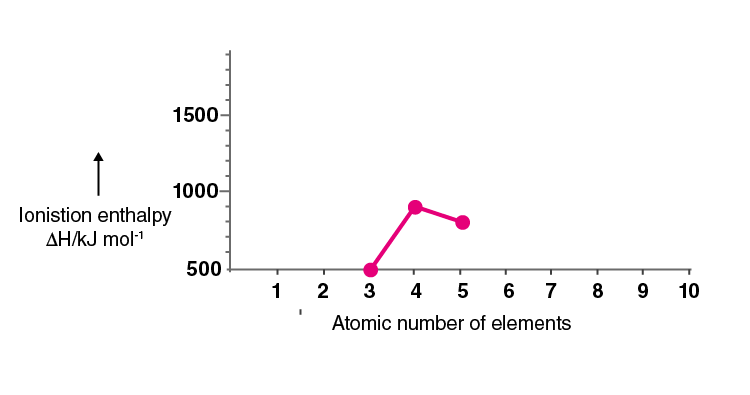Solution: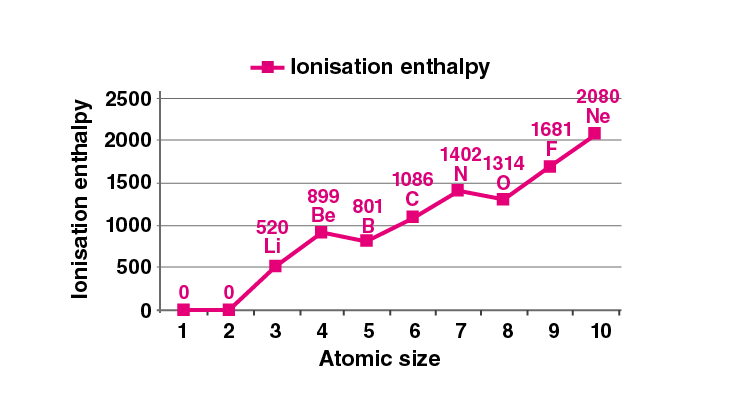The ionisation enthalpy of elements varies across period and group. The ionisation enthalpy decreases down a group and increases as we move from left to right in a period.

28. Among the elements B, Al, C and Si,

(i) which element has the highest first ionisation enthalpy?

(ii) which element has the most metallic character? Justify your answer in each case.

Solution:

(i) Carbon has the highest ionisation enthalpy. It increases from left to right across the period and decreases as we go down the group.

(ii) Aluminium has the most metallic character. As we move down, the metallic character increases and decreases across the period from left to right.

29. Write four characteristic properties of p-block elements.

Solution:

1. They show variable oxidation states. The reducing character increases down the group and oxidising character increases along the period.

2. They have a high ionisation enthalpy than the s-block elements.

3. They usually form covalent compounds.

4. Both metals and non-metals are found in this group, but non-metals are slightly more in number.

30. Choose the correct order of atomic radii of fluorine and neon (in pm) out of the options given below and justify your answer.

(i) 72, 160

(ii) 160, 160

(iii) 72, 72

(iv) 160, 72

Solution:

(i) 72, 160

Because neon has van der Waals radii and fluorine has covalent radii. Covalent radius is always less than van der Waal’s radius, so the radius of Fluorine is 72pm and Neon is 160pm.

31. Illustrate by taking examples of transition elements and non-transition elements that oxidation states of elements are largely based on electronic configuration.

Solution:

Ti has an atomic number of 22 and electronic configuration [Ar]3d24s2 and can show three oxidation states of +2,+3 and +4 in various compounds like TiO29(+4), Ti2O3(+3) and TiO(+2). The non-transition elements like the p block elements show variable oxidation states like in case of phosphorous. It has -3,+3 and +5.

32. Nitrogen has positive electron gain enthalpy whereas oxygen has negative. However, oxygen has lower ionisation enthalpy than nitrogen. Explain.

Solution:

The ionisation enthalpy of oxygen is lower than that of Nitrogen as because when we remove one electron from oxygen then it easily donates it to attain half-filled stability but in case of nitrogen, it is difficult to remove one electron as it already has half-filled stability and it would become unstable after that.

33. The first member of each group of representative elements (i.e., s and p-block elements) shows anomalous behaviour. Illustrate with two examples.

Solution:

Lithium and beryllium are examples. Li is the first group element. It has different properties and forms of covalent compounds and nitrides. Beryllium is the first element of the second group. It has various anomalies like it forms a covalent compound with coordination number four, unlike other elements that have a coordination number 6.

34. p-Block elements form acidic, basic and amphoteric oxides. Explain each property by giving two examples and also write the reactions of these oxides with water.

Solution:

ACIDIC OXIDES

SO2, B2O3 are acidic oxides and p block elements.

The reaction of SO2 with water

SO2 + H2O → H2SO3

The reaction of B2O3 with water

B2O3 +3 H2O → 2H3BO3

Acidic oxides are those oxides that form acids after reacting with water.

BASIC OXIDES

Cao, BaO, Ti2O form basic oxides

The reaction of Ti2O with water.

BaO + H2O → Ba(OH)2

REACTION OF CaO WITH WATER

Cao + H2O → Ca(OH)2

Basic oxides are those oxides that form bases after reacting with water

AMPHOTERIC OXIDES

Zinc oxides and aluminium oxides are the two amphoteric oxides.

Reaction of ZnO with water:

ZnO + 2H2O + 2NaOH → Na3Zn[OH]4 + H2

ZnO +2HCl → ZnCl2 + H2O

Reaction of Al2O3 with water:

Al2O3 (s) + 6 NaOH(aq) + 3H2O(l) → Na3 [Al(OH)6] (aq)

Al2O3 (s) + 6HCl(aq) + 9H2O(l) → 2[Al(H2O)]3+(aq) + 6Cl-

35. How would you explain the fact that first ionisation enthalpy of sodium is lower than that of magnesium but its second ionisation enthalpy is higher than that of magnesium?

Solution:

Sodium attains stable configuration when it loses on an electron from its outermost shell. That is why its first ionisation enthalpy is less than magnesium. But in case of second ionisation enthalpy magnesium has one electron in its outermost shell and to attain stability it loses that easily as compared to sodium which is already stable.

36. What do you understand by exothermic reaction and endothermic reaction? Give one example of each type.

Solution:

Exothermic reaction- The reaction in which heat is evolved is called an exothermic reaction.

for example,

Cao + CO2→ CaCO3 ΔH=-178kJmol-1

Endothermic reaction- The reaction in which heat is absorbed is called an endothermic reaction.

for example,

2NH3 → 3N2+ H2 ΔH=918kJmol-1

37. Arrange the elements N, P, O and S in the order of-

(i) increasing first ionisation enthalpy.

(ii) increasing non-metallic character.

Give the reason for the arrangement assigned.

Solution:

(i) S< P< O< N is the increasing order of the first ionisation enthalpy.

As we go down the group the ionisation enthalpy decreases and as we go along the period then it increases but in case of oxygen and nitrogen due to half-filled stability of 2 p orbitals of nitrogen it has a higher ionisation enthalpy than oxygen.

(ii) P<S<N<O is the increasing order of non-metallic character.

As we go down the group non-metallic character decreases as the effective nuclear charge on the outermost shell decreases which helps to gain an electron. As we move along the period then effective nuclear charge increases which increase the non-metallic character.

38. Explain the deviation in ionisation enthalpy of some elements from the general trend by using Fig. 3.2.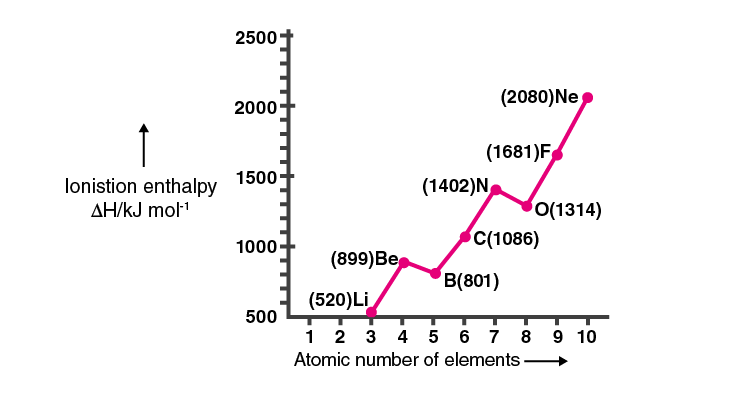Solution:

The ionisation enthalpy of elements varies across period and group. The ionisation enthalpy decreases down a group and increases as we move from left to right in a period.

• Effective nuclear charge on the outermost electrons.

• Electron-electron repulsion force.

• Stability of the element due to half-filled and filled orbitals are some of the parameter affected.

39. Explain the following:

(a) Electronegativity of elements increases on moving from left to right in the periodic table.

(b) Ionisation enthalpy decrease in a group from top to bottom?

Solution:

(a) As we move from left to right in a period, the size of the atoms decreases due to the increase in the effective nuclear charges on the outermost electron. As a result of which electronegativity of elements increases on moving from left to right in the periodic table.

(b) As we go down the group the atomic size increases which result in the increase in the distance of the electrons in the outermost shell as a result of which effective nuclear charge decreases. This results in a decrease of ionisation enthalpy.

40. How does the metallic and non-metallic character vary on moving from left to right in a period?

Solution:

Metallic character decreases when we move from left to right across the period and non-metallic character increases as there is an increase in ionisation enthalpy and electron gain enthalpy along the period.

41. The radius of Na+ cation is less than that of Na atom. Give reason.

Solution:

Sodium atom loses one electron to form sodium cation and after the formation of a cation, the effective nuclear charge on the ion increases on the left electrons which results in a decrease in radius.

42. Among alkali metals which element do you expect to be least electronegative and why?

Solution:

Caesium is the least electronegative alkali metal as electronegativity decreases as we go down the group. Caesium is a group 1 element and lies down the group as it has the largest size due to a decrease in the effective nuclear charge.

IV. Matching Type

43. Match the correct atomic radius with the element.

Solution:44. Match the correct ionisation enthalpies and electron gain enthalpies of the following elements.

Solution:

(i) is B(ii) is A(iii) is D(iv) is C

45. Electronic configuration of some elements is given in Column I and their electron gain enthalpies are given in Column II. Match the electronic configuration with electron gain enthalpySolution: V. Assertion and Reason TypeIn the following questions, a statement of Assertion (A) followed by a statement of the reason (R) is given. Choose the correct option out of the choices given below each question.46. Assertion (A): Generally, ionisation enthalpy increases from left to right in a period.Reason (R): When successive electrons are added to the orbitals in the same principal quantum level, the shielding effect of the inner core of electrons does not increase very much to compensate for the increased attraction of the electron to the nucleus. (i) The assertion is correct statement and reason is the wrong statement. (ii) Assertion and reason both are correct statements and reason is the correct explanation of assertion. (iii) Assertion and reason both are wrong statements. (iv) The assertion is wrong statement and reason is the correct statement.

Solution:

Option (ii) is correct.

47. Assertion (A): Boron has a smaller first ionisation enthalpy than beryllium.Reason (R): The penetration of a 2s electron to the nucleus is more than the 2p electron hence 2p electron is more shielded by the inner core of electrons than the 2s electrons. (i) Assertion and reason both are correct statements but the reason is not the correct explanation for the assertion. (ii) The assertion is a correct statement but the reason is the wrong statement. (iii) Assertion and reason both are correct statements and reason is the correct explanation for the assertion. (iv) Assertion and reason both are wrong statements.

Solution:

Option (iii) is correct.

48. Assertion (A): Electron gain enthalpy becomes less negative as we go down a group.Reason (R): Size of the atom increases on going down the group and the added electron would be farther from the nucleus. (i) Assertion and reason both are correct statements but the reason is not the correct explanation for the assertion. (ii) Assertion and reason both are correct statements and reason is the correct explanation for the assertion. (iii) Assertion and reason both are wrong statements. (iv) The assertion is the wrong statement but the reason is the correct statement.

Solution:

Option (iv) is correct.

49. Discuss the factors affecting electron gain enthalpy and the trend in its variation in the periodic table.

Solution;

The factors affecting electron gain enthalpy and the trend in its variation in the periodic table are:

1)ATOMIC SIZEAs we go down the group electron gain enthalpy decreases as the distance of the nucleus from the outermost shell increases which decreases its tendency to gain electron and electron gain enthalpy becomes less negative.

2)EFFECTIVE NUCLEAR CHARGEAs we go from left to right in a period the effective nuclear charge increases and when we move down the group it decreases which results in the attraction of electrons from the outermost shell

3) ELECTRONIC CONFIGURATIONThe tendency to gain electrons depends upon the stability of an element. Elements having completely or half-filled stable orbitals have a very low tendency to gain electron thus they have very low electron gain enthalpy.TRENDSAcross a period the electron gain enthalpy becomes more negative. Down the group, the electron gain enthalpy becomes less negative.

50. Define ionisation enthalpy. Discuss the factors affecting ionisation enthalpy of the elements and its trends in the periodic table.

Solution:

Ionisation enthalpy is the energy required by an isolated and gaseous atom in its ground state to remove an electron. The effective nuclear charge due to the screening effect, the valence electrons are shielded by the inner core electrons. This effective nuclear charge is less than the actual charge present on the atom. Penetrated orbitals: It is difficult to remove an electron from the orbitals that are closer to the nucleus and are penetrated towards the nucleus. The order of penetration is given by s>p>d>fStability of orbitals: Half-filled and filled orbitals have a high ionisation enthalpy as they don’t want to lose their stability. Across a period the ionisation enthalpy increases along the period. Down the group, the ionisation enthalpy decreases.

Important Concepts of Class 11 Chemistry Chapter 3 Classification of Elements

• Why Do We Need to Classify Elements?
• Genesis of Periodic Classification
• Modern Periodic Law and the Present Form of the Periodic Table
• Nomenclature of Elements with Atomic Numbers > 100
• Electronic Configurations of Elements and the Periodic Table
• Electronic Configurations and Types of Elements: 5-, P-, D-, F- Blocks Ex
• The S-block Elements
• The P-block Elements
• The D-block Elements (Transition Elements)
• The F-block Elements (Inner-transition Elements)
• Metals, Non-metals and Metalloids
• Periodic Trends in Properties of Elements
• Trends in Physical Properties
• Periodic Trends in Chemical Properties
• Periodic Trends and Chemical Reactivity

BYJU’S presents innovative learning opportunities for students studying in Class 11 and Class 12. BYJU’S provides you with video lessons to make learning fun for you. Our innovative teaching methods will make you fall in love with learning.

## Frequently Asked Questions on NCERT Exemplar Solutions for Class 11 Chemistry Chapter 3

Q1

### List out the topics discussed in Chapter 3 of NCERT Exemplar Solutions for Class 11 Chemistry.

Important concepts of Class 11 Chemistry Chapter 3 Classification of Elements are listed below.
1. Why Do We Need to Classify Elements?
2. Genesis of Periodic Classification
3. Modern Periodic Law and the Present Form of the Periodic Table
4. Nomenclature of Elements with Atomic Numbers > 100
5. Electronic Configurations of Elements and the Periodic Table
6. Electronic Configurations and Types of Elements: 5-, P-, D-, F- Blocks Ex
7. The S-block Elements
8. The P-block Elements
9. The D-block Elements (Transition Elements)
10. The F-block Elements (Inner-transition Elements)
11. Metals, Non-metals and Metalloids
12. Periodic Trends in Properties of Elements
13. Trends in Physical Properties
14. Periodic Trends in Chemical Properties
15. Periodic Trends and Chemical Reactivity
Q2

### How should I prepare Chapter 3 of NCERT Exemplar Solutions for Class 11 Chemistry for the final exam?

Studying this chapter regularly  will help you to get a grip on the concepts. Taking notes about complex topics and difficult numericals will boost your confidence during revision. After completing the questions from the NCERT exemplar textbook, you can refer to the solutions prepared by the expert faculty at BYJU’S to understand a different method of answering the questions. The solutions contain detailed explanations for all topics, which are important for the final exam.
Q3

### Why should I download the NCERT Exemplar Solutions for Class 11 Chemistry Chapter 3 PDF from BYJU’S?

Students can download the NCERT Exemplar Solutions for Class 11 Chemistry Chapter 3 PDF from BYJU’S. The solutions created are 100% accurate based on the CBSE syllabus and guidelines. Students can find the PDF format of solutions, which are free for download on BYJU’S. Chapter-wise solutions are available to help students boost their exam preparation.
 Element Be C O B N Atomic radius (pm) 74 88 111 77 66 Element Be C O B N Atomic radius (pm) 111 77 66 88 74 Elements (i) Most reactive non-metal (ii) Most reactive metal iii) Least reactive element (iv) Metal forming binary halide ∆ H1 A. 419 B. 1681 C. 738 D. 2372 ∆ H2 3051 3374 1451 5251 ∆eg H -48 -328 -40 +48 Column (I) Electronic configuration (i) 1s2 2s2 sp6 (ii) 1s2 2s2 2p6 3s1 (iii) 1s2 2s2 2p5 (iv) 1s2 2s2 2p4 Column (II) Electron gain enthalpy/kJ mol–1 (A) – 53 (B) – 328 (C) – 141 (D) + 48 Column (I) Electronic configuration (i) 1s2 2s2 sp6 (ii) 1s2 2s2 2p6 3s1 (iii) 1s2 2s2 2p5 (iv) 1s2 2s2 2p4 Column (II) Electron gain enthalpy/kJ mol–1 (D) +48 (A) -53 (B) -328 (C)-141 Also Access NCERT Solutions for Class 11 Chemistry Chapter 3 CBSE Notes for Class 11 Chemistry Chapter 3